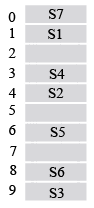# ISRO Scientist or Engineer Computer Science 2018

Instructions

For the following questions answer them individually

Question 61

# Consider the following declaration :structaddr{ char city; char street; int pin ;};struct { char name ; int gender ; struct addr locate ;}person, *kd = & person;Then $$*(kd \rightarrow name +2)$$ can be used instead ofQuestion 62

# If a variable can take only integral values from 0 to n, where n is an integer, then the variable can be represented as a bit-field whose width is ( the log in the answersare to the base 2, and [log n] means the floor of logn )Question 63

# The following C programmain(){ fork() ; fork() ; printf("yes"); }If we execute this core segment, how manytimes the string yes will be printed?Question 64

# Given $$\sqrt{224_r} = 13_r$$ the value of radix r isQuestion 65

# Determine the number of page faults when references to pages occur in the order - 1, 2, 4, 5, 2, 1, 2, 4. Assume that the main memory can accommodate 3 pages and the main memory already has the pages 1 and 2, with page 1 having brought earlier than page 2. (assume LRU algorithm is applied)Question 66

# Consider a system having m resources of the same type. These resources are started by 3 processes A, B, C, which have peak time demands of 3, 4, 6 respectively. The minimum value of m that ensures that deadlock will never occur isQuestion 67

# A computerhas 1000K of main memory. The jobs arrive and finish in the following sequence Job 1 requiring 200K arrivesJob 2 requiring 350K arrivesJob 3 requiring 300K arrivesJob 1 finishesJob 4 requiring 120K arrivesJob5 requiring 150K arrivesJob 6 requiring 80K arrivesAmong best fit andfirst fit, which performs better for this sequence?Question 68

# Disk requests cometo a disk driver for cylinders in the order 10, 22, 20, 2, 40, 6 and 38, at a time when the disk drive is reading from cylinder 20. The seek time is 6 ms/cylinder. The total seek time, if the disk arm scheduling algorithms is first-come-first-served isQuestion 69

# A has table with 10 buckets with one slot per bucket is depicted here. The symbols, S1 to S7 are initially entered using a hashing function with linear probing. The maximum number of comparisons needed in searching an item that is not present isQuestion 70

# The running time of an algorithm is given byT(n) = T(n-1) + T(n-2) - T(n-3), if n > 3 = n, otherwiseThen what should be the relation between T(1), T(2) and T(3), so that the order of the algorithm is constant ?OR